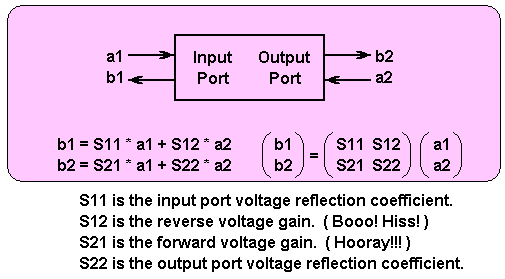# The y-, z-, h-, and s-parameter alphabet soupThe analysis of two-port networks is a topic well covered in literature. Such analyses start looking a little like alphabet soup when we speak, for example, of y-parameters, z-parameters, and h-parameters. Those three are summed up for the simplest cases as follows in Figure 1.

Figure 1 Two-port y-parameters (green), z-Parameters (yellow), and h-Parameters (blue).

For the y-parameters, each coefficient is an admittance. For the z-parameters, each coefficient is an impedance. For the h-parameters, we have an impedance for h11, an admittance for h22 and two dimensionless terms h12 and h21, from which a terminology mix leads to these being called hybrid parameters.

The governing algebra is shown for each case along with the corresponding matrix notation. In all cases, the coefficients are allowed to be complex numbers with real and imaginary parts.

It can sometimes be difficult to assess the y, z and h values in the real world, especially when we get to higher frequencies. An easily visualized example of that difficulty is z11 = V1 / I1 when I2 = zero, but actually making I2 be zero is not necessarily all that easy. How would you accomplish that if the two-port device under examination is a waveguide? Similarly, how would you examine h11 = V1 / I1 when V2 = zero? Do you know how to make a true short circuit across a microwave structure? I don’t.

A way out of this issue is to use s-parameters as shown in Figure 2.Figure 2 The two-port s-parameter matrix (violet).

What actually appears at port 1 is the sum of voltages a1 and b1 while what appears at Port 2 is the sum of a2 and b2. What makes this useful is that the s-parameter numbers are defined for the network operating at a termination impedance, in many cases, at 50 Ω. All of the s-parameters are dimensionless coefficients which, as before, can be complex numbers. Those coefficients can also vary with frequency as well.

S-parameters are often called “scattering parameters” and their matrix is a “scattering matrix”. There is lots of literature about that, so I won’t try to go into that here. However, I once read a story, perhaps apocryphal, which I thought was pretty cool stuff.

If we have a two-port device of some kind in which every last little bit of structure complies perfectly with some characteristic impedance and we load port 2 of that structure with exactly that characteristic impedance, then all of the power and energy that enters port 1 will be delivered to the load on port 2 with none of that energy getting bounced back toward its source. For example, if the load impedance being fed by port 2 is exactly the characteristic impedance of the apparatus, the a2 value will  be zero.

However, if there is any structural anomaly along the way, maybe some small metal dimple or some teeny, little edge where maybe two sections of waveguide meet, some small portion of energy will be reflected back from whence it came. That reflection process arises from “scattering” of the signal when and where it meets that little anomaly. Hence the term “scattering parameters”.

True? False? I don’t know. Fun though.

John Dunn is an electronics consultant, and a graduate of The Polytechnic Institute of Brooklyn (BSEE) and of New York University (MSEE).

Related Content

Scroll to Top